Function Repository Resource:

# InteractiveConicPlot

Display an interactive plot with all relevant information for a given conic section

Contributed by: Wolfram|Alpha Math Team
 ResourceFunction["InteractiveConicPlot"][expr,{x,y}] returns an interactive display of the properties of the conic section given by expr in terms of the variables x and y.

## Details

The input expr can be an equation or an Association of properties for a conic section in terms of the variables x and y.
The three distinct, non-degenerate conic sections are parabolas, hyperbolas and ellipses, with circles as a special case of an ellipse.
Parabola properties returned are "Equation", "Focus ", "Vertex", "Directrix", "FocalParameter", "SemiAxisLength", "AxisOfSymmetry", "SemiLatusRectum", "RotationAngle" and "BaseConic".
Ellipse properties returned are "Equation", "Foci", "Vertices", "Covertices", "Eccentricity", "Center", "SemimajorAxisLength", "SemiminorAxisLength", "AreaEnclosed", "SemiLatusRectum", "Directrices", "AxesOfSymmetry", "Circumference", "RotationAngle" and "BaseConic".
Circle properties returned are "Equation", "Radius", "Circumference", "Center" and "AreaEnclosed".
Hyperbola properties returned are "Equation", "Foci", "Vertices", "Eccentricity", "Asymptotes", "Center", "SemimajorAxisLength", "SemiminorAxisLength", "Directrices", "AxesOfSymmetry", "SemiLatusRectum", "RotationAngle", "ConjugateHyperbola" and "BaseConic".

## Examples

### Basic Examples (2)

Display the properties of a parabola:

 In:=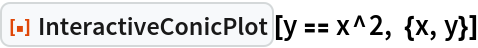Out=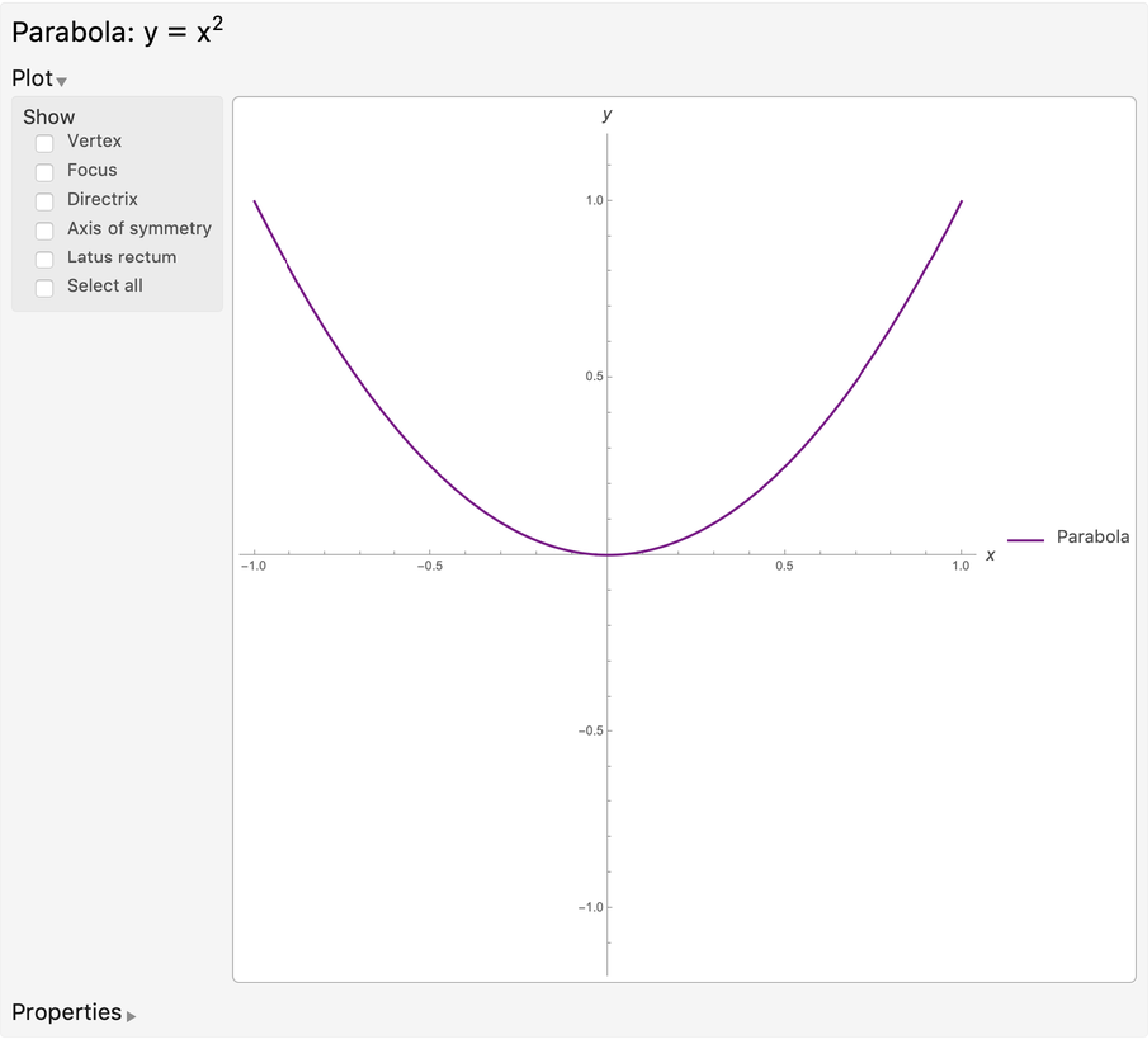Display the properties of a hyperbola:

 In:=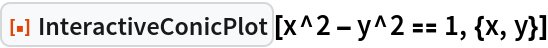Out=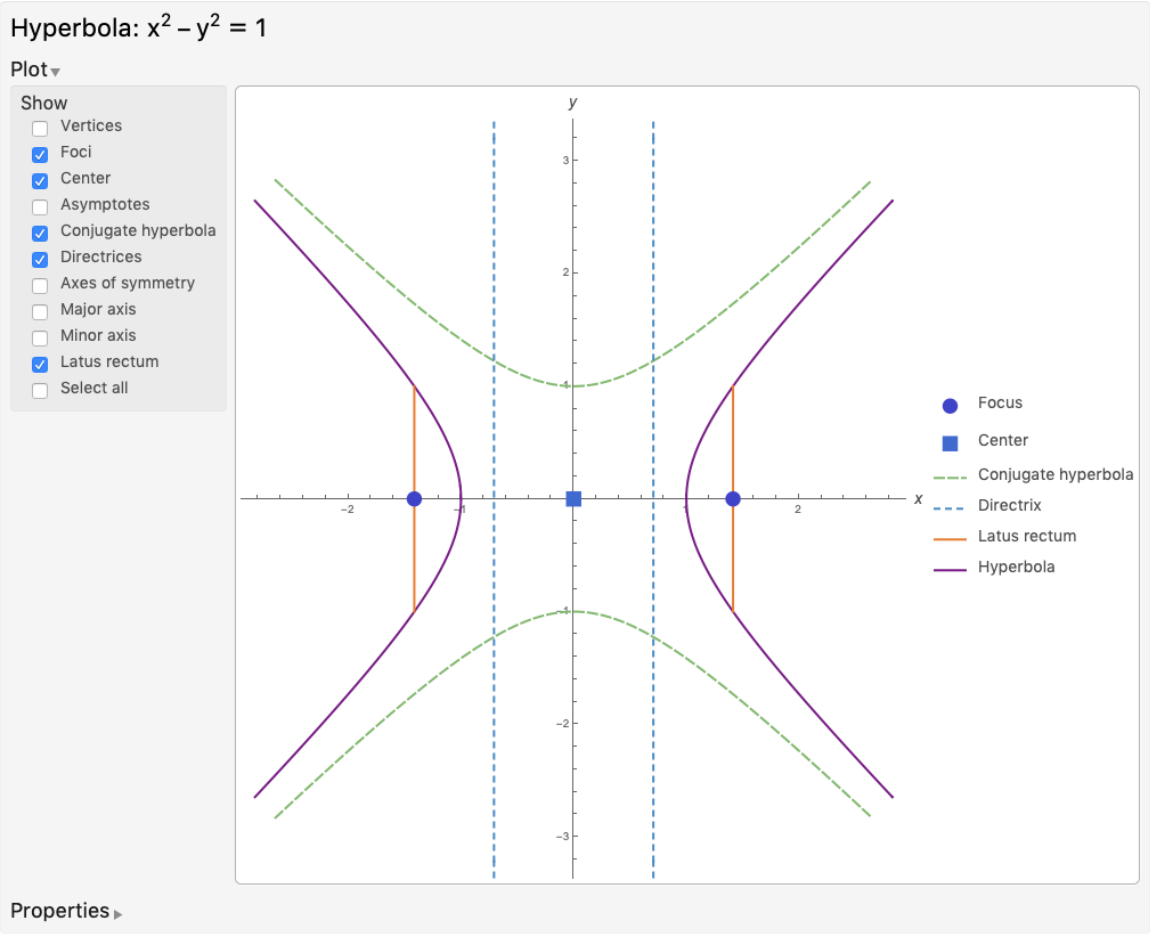### Scope (1)

Provide an association of properties for an unknown conic section and compute the full association of properties, including the type of conic section:

 In:=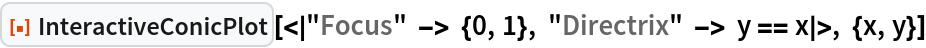Out=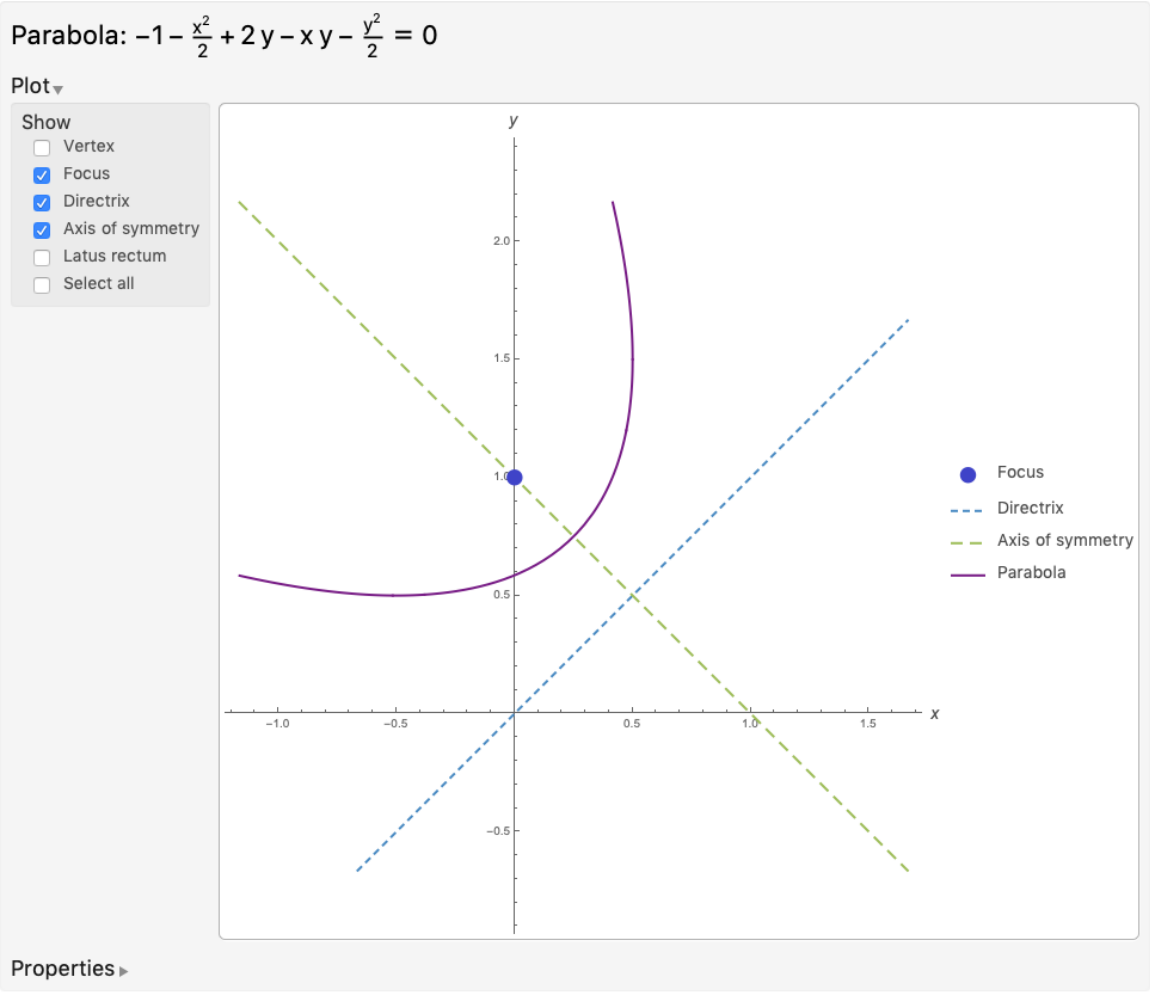### Options (1)

Toggle the "Plot" and "Properties" tabs on or off:

 In:=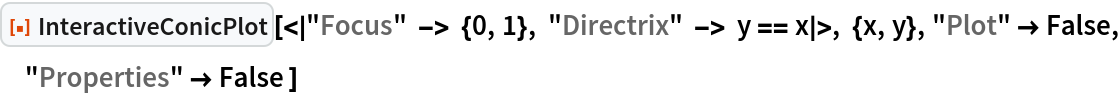Out=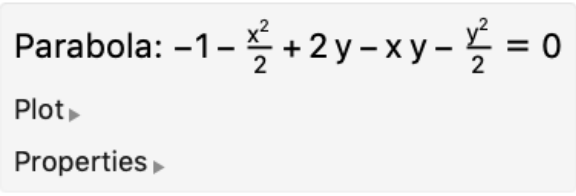### Properties and Relations (1)

The Association of properties displayed by InteractiveConicPlot can be obtained by using the ConicProperties resource function:

 In:=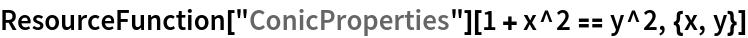Out=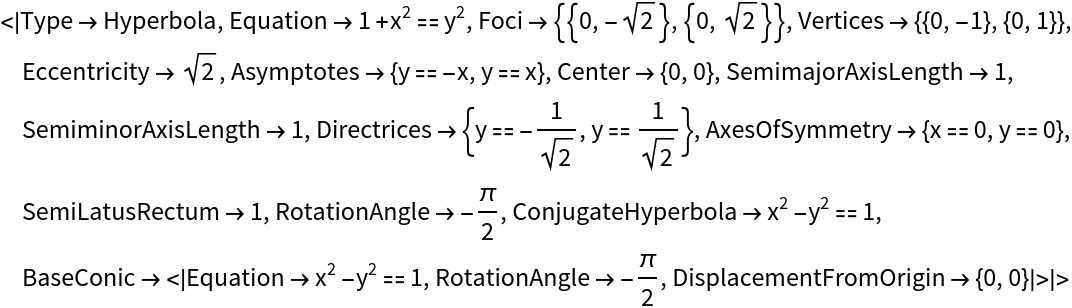### Possible Issues (1)

InteractiveConicPlot does not accept inputs with parameters:

 In:=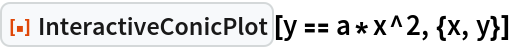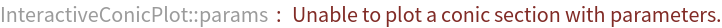Out=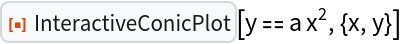## Publisher

Wolfram|Alpha Math Team

## Version History

• 3.0.0 – 23 March 2023
• 2.2.0 – 02 February 2021
• 2.1.0 – 02 February 2021
• 2.0.0 – 01 May 2020
• 1.0.0 – 01 May 2020